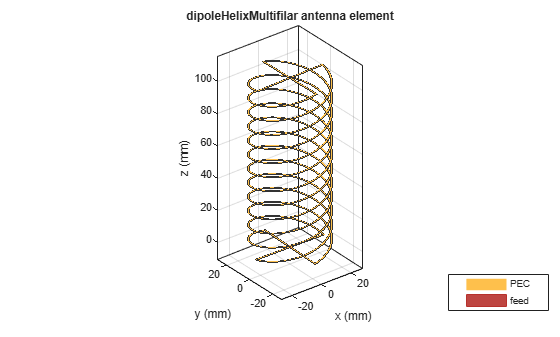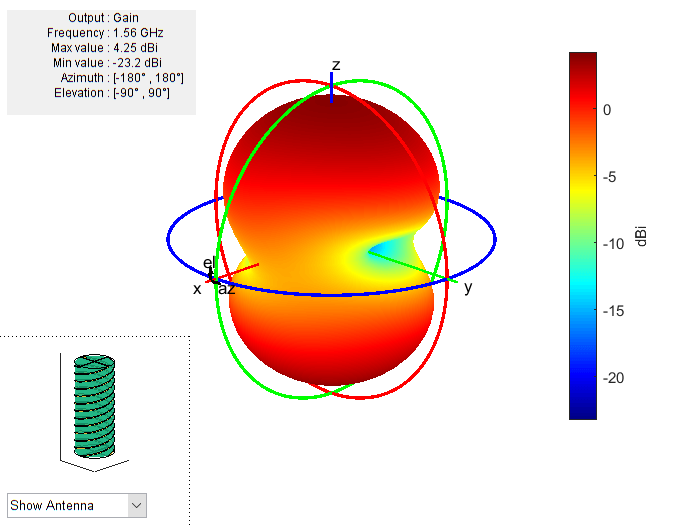# dipoleHelixMultifilar

Create balanced bifilar or quadrafilar dipole helix antenna without circular ground plane

## Description

The `dipoleHelixMultifilar` object creates a balanced bifilar or quadrafilar helix antenna without a circular ground plane. You can create both short-circuited and open-ended dipole helix multifilar antennas. Bifilar and quadrafilar helix antennas are used in aerospace and defense applications.

The width of the strip is related to the diameter of an equivalent cylinder by the equation

`$w=2d=4r$`

where:

• w is the width of the strip.

• d is the diameter of an equivalent cylinder.

• r is the radius of an equivalent cylinder.

For a given cylinder radius, use the `cylinder2strip` utility function to calculate the equivalent width. The default helix antenna is end-fed. The circular ground plane is on the xy- plane. Helix antennas are used commonly in axial mode. In this mode, the helix circumference is comparable to the operating wavelength, and the helix has maximum directivity along its axis. In normal mode, the helix radius is small compared to the operating wavelength. In this mode, the helix radiates broadside, that is, in the plane perpendicular to its axis. The basic equations for the helix are

$\begin{array}{l}x=r\mathrm{cos}\left(\theta \right)\\ y=r\mathrm{sin}\left(\theta \right)\\ z=S\theta \end{array}$

where:

• r is the radius of the helical dipole.

• θ is the winding angle.

• S is the spacing between turns.

For a given pitch angle in degrees, use the `helixpitch2spacing` utility function to calculate the spacing between the turns in meters.## Creation

### Syntax

``ant = dipoleHelixMultifilar``
``ant = dipoleHelixMultifilar(Name=Value)``

### Description

example

````ant = dipoleHelixMultifilar` creates a bifilar or quadrafilar helix antenna without a circular ground plane. The default multifilar helical antenna is end-fed and operates around 2 GHz.```

example

````ant = dipoleHelixMultifilar(Name=Value)` creates a multifilar helical dipole antenna, with additional Properties specified by one or more name–value arguments. `Name` is the property name and `Value` is the corresponding value. You can specify several name-value arguments in any order as `Name1`= `Value1`, `...`, `NameN`=`ValueN`. Properties not specified retain their default values.For example, `ant = dipoleHelixMultifilar(Radius=28e-03)` creates a multifilar helix with turns of radius `28e-03` m.```

## Properties

expand all

Number of helical elements, specified as a `4` or `2`. Two elements create a bifilar dipole helix antenna, and four elements create a quadrafilar dipole helix antenna.

Example: `NumArms=2`

Data Types: `double`

Radius of the turns, specified as a positive real scalar meter.

Example: `Radius=28e-03`

Data Types: `double`

Width of the strip, specified as a positive real scalar in meters.

Example: `Width=0.2`

Data Types: `double`

Number of turns, specified as a scalar integer.

Example: `Turns=4`

Data Types: `double`

Spacing between the turns, specified as a positive real scalar in meters.

Example: `Spacing=7.5e-2`

Data Types: `double`

Status of ends of the helix, specified as `0` or `1`. By default, the `dipoleHelixMultifilar` is short circuited. Setting the property to `0` makes the helix antenna an open circuit.

Example: `ShortEnds=0`

Data Types: `double`

Direction of helix turns (windings), specified as `CW` or `CCW`.

Example: `WindingDirection="CW"`

Data Types: `string`

Substrate dielectric material, specified as "air" or a `dielectric` object. You can specify only one dielectric layer in the `dipoleHelixMultifilar` object. Specify the same radius for all the turns. When you use a dielectric material other than air, the number of turns in the dipole helix must be greater than 1. For a short-ended configuration, the number of turns must be a positive integer. For more information about dielectric substrate meshing, see Meshing.

Example: `dielectric("Teflon")`

Data Types: `string`

Type of the metal used as a conductor, specified as a metal material object. You can choose any metal from the `MetalCatalog` or specify a metal of your choice. For more information, see `metal`. For more information on metal conductor meshing, see Meshing.

Example: `metal("Copper")`

Lumped elements added to the antenna feed, specified as a lumped element object. You can add a load anywhere on the surface of the antenna. By default, the load is at the origin. For more information, see `lumpedElement`.

Example: `Load=lumpedelement`. `lumpedelement` is the object for the load created using `lumpedElement`.

Example: `Load=lumpedElement(Impedance=75)`

Data Types: `double`

Tilt angle of the antenna in degrees, specified as a scalar or vector. For more information, see Rotate Antennas and Arrays.

Example: `90`

Example: `Tilt=[90 90]`,`TiltAxis=[0 1 0;0 1 1]` tilts the antenna at 90 degrees about the two axes defined by the vectors.

Data Types: `double`

Tilt axis of the antenna, specified as one of these values:

• Three-element vector of Cartesian coordinates in meters. In this case, each coordinate in the vector starts at the origin and lies along the specified points on the x-, y-, and z-axes.

• Two points in space, specified as a 2-by-3 matrix corresponding to two three-element vectors of Cartesian coordinates. In this case, the antenna rotates around the line joining the two points.

• `"x"`, `"y"`, or `"z"` to describe a rotation about the x-, y-, or z-axis, respectively.

Example: `[0 1 0]`

Example: `[0 0 0;0 1 0]`

Example: `"Z"`

Data Types: `double` | `string`

## Object Functions

 `show` Display antenna, array structures, shapes, or platform `axialRatio` Axial ratio of antenna `beamwidth` Beamwidth of antenna `charge` Charge distribution on antenna or array surface `current` Current distribution on antenna or array surface `design` Design prototype antenna or arrays for resonance around specified frequency or create AI-based antenna from antenna catalog objects `efficiency` Radiation efficiency of antenna `EHfields` Electric and magnetic fields of antennas or embedded electric and magnetic fields of antenna element in arrays `impedance` Input impedance of antenna or scan impedance of array `mesh` Mesh properties of metal, dielectric antenna, or array structure `meshconfig` Change meshing mode of antenna, array, custom antenna, custom array, or custom geometry `optimize` Optimize antenna or array using SADEA optimizer `pattern` Plot radiation pattern and phase of antenna or array or embedded pattern of antenna element in array `patternAzimuth` Azimuth plane radiation pattern of antenna or array `patternElevation` Elevation plane radiation pattern of antenna or array `rcs` Calculate and plot radar cross section (RCS) of platform, antenna, or array `returnLoss` Return loss of antenna or scan return loss of array `sparameters` Calculate S-parameters for antennas and antenna arrays `vswr` Voltage standing wave ratio (VSWR) of antenna or array element

## Examples

collapse all

Create and view a default multifilar helical dipole antenna.

`ant = dipoleHelixMultifilar`
```ant = dipoleHelixMultifilar with properties: NumArms: 4 Radius: 0.0220 Width: 1.0000e-03 Turns: 3 Spacing: 0.0350 ShortEnds: 1 WindingDirection: 'CCW' Substrate: [1x1 dielectric] Conductor: [1x1 metal] Tilt: 0 TiltAxis: [1 0 0] Load: [1x1 lumpedElement] ```
`show(ant)`Create and view a quadrafilar helical dipole antenna with turn radius of 22 mm and strip width of 1 mm.

`ant = dipoleHelixMultifilar(Radius=22e-3,Width=1e-3);`

Add a Teflon dielectric substrate to it.

```d = dielectric("Teflon"); ant.Substrate = d; show(ant)```Plot the radiation pattern of the helical dipole at 1.56 GHz.

`pattern(ant,1.56e9);`## Version History

Introduced in R2018b